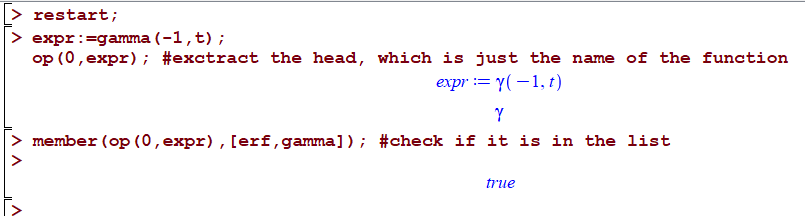# How to check if function name is in a list?

sagemath 8.9

I am still not good in sagemath and learning it. I am trying to convert this code from Maple to sagemath, but I do not know why it is not working in sagemath.

Given a function, I need to find if it is a member of list of known functions. This is easier explained with an example.

Say the input is gamma(-1,t) and I need to find if the function, which is gamma in this case in member of a list of names of functions, such as [erf,gamma,cos, etc...]. I will show the Maple code and how I translated to sagemath. But in sagemath it gives false instead of true.

Maple:

restart;
expr:=gamma(-1,t);
op(0,expr); #extract the head, which is just the name of the function
gamma
member(op(0,expr),[erf,gamma]); #check if it is in the list
trueThis is in sagemath

sage: var('t')
sage: from sage.all import *
sage: expr=gamma(-1,t)
sage: expr.operator()
gamma
sage: expr.operator() in [erf,gamma]
False


So I must be doing something wrong in sagemath. It might be due to type, because when I do

sage: type(expr.operator())
<class 'sage.functions.gamma.Function_gamma_inc'>


Do I need to import something to make it work? I think the name gamma inside the list is not taken as the same as the name gamma which results from expr.operator() and that is why it gives False. But do not know how to fix it.

The idea is to check if the function is among a list of known function names in a list. How to do this in sagemath?

Thanks --Nasser

edit retag close merge delete

Sort by » oldest newest most voted

Hello, @Nasser! Yes, indeed, it seems that the function you get with expr.operator() and gamma are different. I really don't know why. This is the first time I notice this. Actually, if you type:

type(expr.operator())
type(gamma)
type(gamma(t).operator())


you get three different results! In this case,

<class 'sage.functions.gamma.Function_gamma_inc'>
<class 'function'>
<class 'sage.functions.gamma.Function_gamma'>


So, my guess is they are the same function, but with different implementations and, consequently, different data types. This doesn't seem to be a bug, but an unfortunate consequence of the implementation itself. Maybe you should ask about these differences in a separate question in order to get an answer from somebody more experienced and Sage-savvy than me.

If it's all the same to you, you could solve this situation by using strings:

expr = gamma(-1, t)
str(expr.operator()) in ['erf', 'gamma']


That should return True.

Another, more elegant approach, is to use the name() function, but that also reduces to using strings:

expr = gamma(-1, t)
expr.operator().name() in ['erf', 'gamma']

more